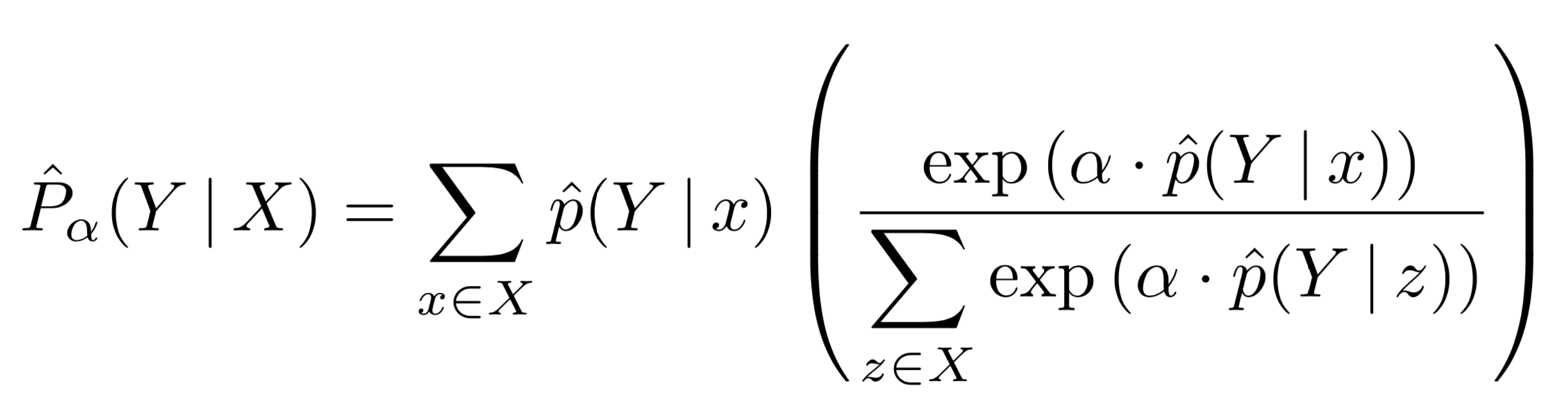# marl/autopool

Adaptive pooling operators for multiple instance learning
Latest commit 4c3e172 Aug 8, 2018
Type Name Latest commit message Commit time
Failed to load latest commit information.autopooldocs.gitignoreLICENSEREADME.mdsetup.py

# autopool

Adaptive pooling operators for Multiple Instance Learning (documentation).

AutoPool is an adaptive (trainable) pooling operator which smoothly interpolates between common pooling operators, such as min-, max-, or average-pooling, automatically adapting to the characteristics of the data.

AutoPool can be readily applied to any differentiable model for time-series label prediction. AutoPool is presented in the following paper, where it is evaluated in conjunction with convolutional neural networks for Sound Event Detection:

Adaptive pooling operators for weakly labeled sound event detection
Brian Mcfee, Justin Salamon, and Juan Pablo Bello
IEEE Transactions on Audio, Speech and Language Processing, In press, 2018.

## Installation

``````pip install autopool
``````

## Definition

AutoPool extends softmax-weighted pooling by adding a trainable parameter α to be learned jointly with all other trainable model parameters:Here, `p(Y|x)` denotes the prediction for an instance `x`, and `X` denotes a set (bag) of instances. The aggregated prediction `P(Y|X)` is a weighted average of the instance-level predictions. Note that when α = 0 this reduces to an unweighted mean; when α = 1 this simplifies to soft-max pooling; and when α → ∞ this approaches the max operator. Hence the name: AutoPool.

## Usage

AutoPool is implemented as a keras layer, so using it is as straightforward as using any standard Keras pooling layer, for example:

``````bag_pred = AutoPool(axis=1)(instance_pred)
``````

Further details and examples are provided in the documentation.

## Constrained and Regularized AutoPool

In the paper we show there can be benefits to either constraining the range α can take, or, alternatively, applying l2 regularization on α; this results in constrained AutoPool (CAP) and regularized AutoPool (RAP) respectively. Since AutoPool is implemented as a keras layer, CAP and RAP can be can be achieved through the layer's optional arugments:

CAP with non-negative α:

``````bag_pred = AutoPool(axis=1, kernel_constraint=keras.constraints.non_neg())(instance_pred)
``````

CAP with α norm-constrained to some value `alpha_max`:

``````bag_pred = AutoPool(axis=1, kernel_constraint=keras.constraints.max_norm(alpha_max, axis=0))(instance_pred)
``````

Heuristics for determining sensible values of `alpha_max` are given in the paper (section III.E).

RAP with l2 regularized α:

``````bag_pred = AutoPool(axis=1, kernel_regularizer=keras.regularizers.l2(l=1e-4))(instance_pred)
``````

CAP and RAP can be combined, of course, by applying both a kernel constraint and a kernel regularizer.

## Multi-label

AutoPool directly generalizes to multi-label settings, in which multiple class labels may be applied to each instance x (for example "car" and "siren" may both be present in an instance). In this setting, a separate auto-pooling operator is applied to each class. Rather than a single parameter α, there is a vector of parameters α_c where c indexes the output vocabulary. This allows a jointly trained model to adapt the pooling strategies independently for each category. Please see the paper for further details.

You can’t perform that action at this time.